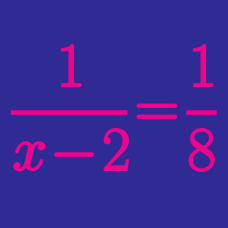Algebra

# Approximation of Square Roots

Which of the following is the closest to $\sqrt{7}?$

Round $\sqrt{137}$ to the nearest integer.

Given $a=2\sqrt{8}, b=2\sqrt{10}, c=\sqrt{35}, d=6,$ and $e=2\sqrt{9},$ which of the following is correct?

Which of the following is the closest to $8.3?$

Which of the following is closest to $2\sqrt{3}?$

×